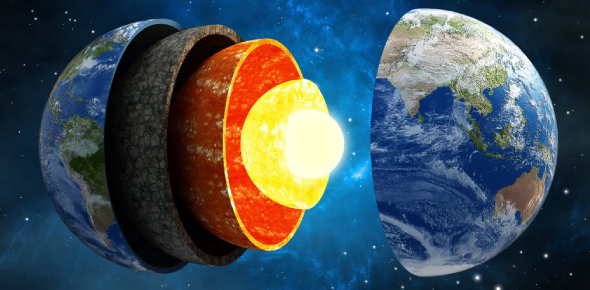# A Quiz On Earth Science! MCQ Trivia

15 Questions | Attempts: 370SettingsIf there is one way that you can successfully revise for a subject, it is using quizzes. I have prepared a series of tests that are designed to help you with passing the earth science exam. Why don’t you take up the test mentioned below and see if you have what it takes to ace the upcoming exam? All the best!

• 1.
What mineral has the chemical formula PbS?
• A.

Graphite

• B.

• C.

Galena

• D.

Malachite

• 2.
How much of an iceberg appears above the water line?
• A.

1/4th

• B.

1/9th

• C.

1/100th

• D.

2-Jan

• 3.
How much salt is in every gallon of seawater?
• A.

32 Pounds Of Salt

• B.

1/18 Pound Of Salt

• C.

1/4 Pound Of Salt

• D.

1 Pound Of Salt

• 4.
The average rain drop falls at a rate of how many feet per second?
• A.

125

• B.

3

• C.

60

• D.

21

• 5.
How many miles long is an average bolt of lightning?
• A.

10

• B.

1

• C.

6

• D.

2

• 6.
How often is the earth hit by lightning?
• A.

100 Times An Hour

• B.

1 Time A Second

• C.

100 Times A Second

• D.

30 Times A Minute

• 7.
What produces more energy than all the world's nuclear weapons combined?
• A.

Lightning

• B.

• C.

Earthquake

• D.

Hurricane

• 8.
What family are both poison oak and poison ivy part of?
• A.

Poison

• B.

Botanical

• C.

Oak

• D.

Cashew

• 9.
How many times does lightning strike the Earth every second?
• A.

700 Times

• B.

2,000 Times

• C.

100 Times

• D.

30 Times

• 10.
What is the test that can help determine the hardness of a rock called?
• A.

Scratch Test

• B.

Malleability Test

• C.

Streak Test

• D.

Density Test

• 11.
Which of the following lakes has a higher salt content than normal?
• A.

Albert Lake

• B.

Lake Erie

• C.

Lake Superior

• D.

Lake Michigan

• 12.
What are large volcanoes that rise from the sea floor called?
• A.

Water Spouts

• B.

Lava Tubes

• C.

Dormant

• D.

Seamounts

• 13.
What submersible helped to discover the wreckage of the Titanic in 1986?
• A.

Alvin

• B.

Atlantis

• C.

Columbus

• D.

Titania

• 14.
What fuel comes from the remains of 300-million-year-old decayed fern plants?
• A.

Coal

• B.

Natural Gas

• C.

Oil

• D.

Gasoline

• 15.
What's the name for the weight of air pushing down on Earth's surface?
• A.

Humidity

• B.

Barometer

• C.

Gravity

• D.

Air Pressure

## Related TopicsBack to top
×

Wait!
Here's an interesting quiz for you.